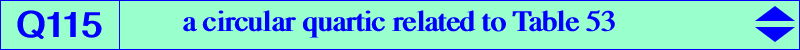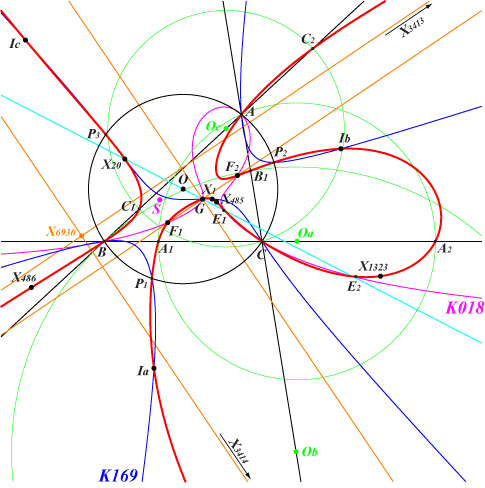too complicated to be written here. Click on the link to download a text file.X(1), X(2), X(20), X(485), X(486), X(1323), X(3413), X(3414), X(5000), X(5001) excenters foci of the Steiner inellipse points P1, P2, P3 of K169 on (O), other cubics below other points belowQ115 is defined in Table 53, paragraph "A net of cubics". Q115 is a circular quartic with singular focus X(187). It meets the line at infinity again at X(3413), X(3414) on the Kiepert hyperbola and the tangents to Q115 at these points meet at X(6390). The tangents to Q115 at : • A, B, C concur at X(3), • the in/excenters concur at X(20), • P1, P2, P3 concur at a point S on the line X(3)X(69) with first barycentric coordinate : a^2 (a^2-b^2-c^2) (a^6-a^4 b^2-a^2 b^4+b^6-a^4 c^2+2 a^2 b^2 c^2-5 b^4 c^2-a^2 c^4-5 b^2 c^4+c^6) and SEARCH = 5.70089851684587. • the foci of the Steiner inellipse concur at a point on the Brocard axis with first bary : a^2 (3 a^6-8 a^4 b^2+9 a^2 b^4-4 b^6-8 a^4 c^2+5 a^2 b^2 c^2-b^4 c^2+9 a^2 c^4-b^2 c^4-4 c^6-2 Sqrt[a^4-a^2 b^2+b^4-a^2 c^2-b^2 c^2+c^4] (a^4-3 a^2 b^2+2 b^4-3 a^2 c^2+b^2 c^2+2 c^4)) and SEARCH = 2.05771500863295. *** Q115 meets the sideline BC again at two points A1, A2 lying on the circle passing through A with center Oa = 0 : a^2-2 b^2 : -a^2+2 c^2. The points B1, B2 and C1, C2 are defined likewise. Q115 meets the Euler line at X(2), X(20) and two other points E1, E2 which lie on K018. These points are X(5000), X(5001) in ETC and they are real if and only if ABC is acutangle. Q115 and Q019 are two very similar quartics meeting at 16 identified points namely A, B, C, the circular points at infinity each counted twice, X(2), X(3413), X(3414), X(5000), X(5001) and the four foci of the Steiner inellipse. They generate a pencil of quartics which contains the degenerate quartic into K018 and the line at infinity and also the quartics Q116, Q117, Q118. *** Other cubics through the points P1, P2, P3 Every pK with pole Ω on K177 and pivot P on K141 passes through the points P1, P2, P3 when Ω is X2 / gtΩ = X6 x P or, equivalently, P = tg(X2 / Ω). Here, X2 / M denotes the X2–Ceva conjugate of M, the center (resp. perspector) of the circum-conic with perspector (resp. center) M. For example, the cubics with pole X2, X3, X6, X32 are K141, K168, K169, K174 respectively. Also pK(X206, X6), pK(3162, X4).